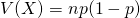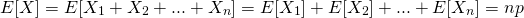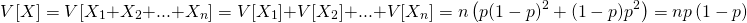# Binomial distribution

Binomial distribution is the discrete probability distribution of the number of successes in a sequence of n independent yes/no experiments, each of which yields success with probability p.

If the random variable X fulfils below with parameters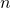and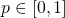, we say that X follows the binomial distribution B(n,p).

We write that as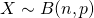.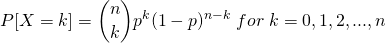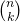is binomial coefficients.

(1)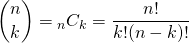We call this distribution as Bernoulli distribution if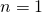If, expected value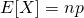, variance